# Indicator Disappears when compiled122

when i compile the indicator it stops working

there is no error in the code only two warnings

its working fine but when i open it in the metaeditor and compile again (with or without making any changes) , it stops working,what could be the problem?

```//+------------+-----------------------------------------------------+
//| v.12.04.07 |                                            SSRC.mq4 |
//+------------+              Spearman,                              |
//|            |              Rosh                                   |
//|            |              È ïðèìàçàâøèéñÿ ê íèì                  |
//|            |              Bookkeeper, 2007, yuzefovich@gmail.com |
//+------------+-----------------------------------------------------+
// http://www.metaquotes.net
// http://codebase.mql4.com/ru/1023
//----
#property indicator_separate_window
#property indicator_maximum  1.3
#property indicator_minimum -1.3
#property indicator_level1   0.9
#property indicator_level2  -0.9
#property indicator_level3   0.8
#property indicator_level4  -0.8
#property indicator_level5   0.7
#property indicator_level6  -0.7
#property indicator_levelcolor DimGray
#property indicator_buffers  1
#property indicator_color1   Maroon
//---- input parameters
//---- Snake
extern int    SnakeRange   =3;
extern int    FilterPeriod =21;
extern double MartFiltr    =2;
extern int    PriceConst   =0; // 0 - Close
// 1 - Open
// 2 - High
// 3 - Low
// 4 - (H+L)/2
// 5 - (H+L+C)/3
// 6 - (H+L+2*C)/4
//---- buffers
double SRCBuffer[];
double Axis[];
double Mart[];
#define SIGNAL_BAR 1

//---- SpearmanRankCorrelation
int    rangeN = 14; //   = 30 maximum
double R2[];
double multiply;
int    PriceInt[];
int    SortInt[];
//+------------------------------------------------------------------+
void MartAxis(int Pos) { int SnakeWeight,i,w,ww,Shift;double SnakeSum;
switch(PriceConst) {
case  0:
Axis[Pos]=iMA(NULL,0,SnakeRange+1,0,MODE_LWMA,PRICE_CLOSE,Pos);
break;
case  1:
Axis[Pos]=iMA(NULL,0,SnakeRange+1,0,MODE_LWMA,PRICE_OPEN,Pos);
break;
case  2:
Axis[Pos]=iMA(NULL,0,SnakeRange+1,0,MODE_LWMA,PRICE_HIGH,Pos);
break;
case  3:
Axis[Pos]=iMA(NULL,0,SnakeRange+1,0,MODE_LWMA,PRICE_LOW,Pos);
break;
case  4:
Axis[Pos]=iMA(NULL,0,SnakeRange+1,0,MODE_LWMA,PRICE_MEDIAN,Pos);
break;
case  5:
Axis[Pos]=iMA(NULL,0,SnakeRange+1,0,MODE_LWMA,PRICE_TYPICAL,Pos);
break;
case  6:
Axis[Pos]=iMA(NULL,0,SnakeRange+1,0,MODE_LWMA,PRICE_WEIGHTED,Pos);
break;
default:
Axis[Pos]=iMA(NULL,0,SnakeRange+1,0,MODE_LWMA,PRICE_WEIGHTED,Pos);
break; }
for(Shift=Pos+SnakeRange+2;Shift>Pos;Shift--) { SnakeSum=0.0;
SnakeWeight=0; i=0; w=Shift+SnakeRange; ww=Shift-SnakeRange;
if(ww<Pos) ww=Pos;
while(w>=Shift) { i++; SnakeSum=SnakeSum+i*SnakePrice(w);
SnakeWeight=SnakeWeight+i; w--; }
while(w>=ww) { i--; SnakeSum=SnakeSum+i*SnakePrice(w);
SnakeWeight=SnakeWeight+i; w--; }
Axis[Shift]=SnakeSum/SnakeWeight; } return; }
//----
double SnakePrice(int Shift) {
switch(PriceConst) {
case  0: return(Close[Shift]);
case  1: return(Open[Shift]);
case  2: return(High[Shift]);
case  3: return(Low[Shift]);
case  4: return((High[Shift]+Low[Shift])/2);
case  5: return((Close[Shift]+High[Shift]+Low[Shift])/3);
case  6: return((2*Close[Shift]+High[Shift]+Low[Shift])/4);
default: return(Close[Shift]); } }
//+------------------------------------------------------------------+
void SmoothOverMart(int Shift) { double t,b;
t=Axis[ArrayMaximum(Axis,FilterPeriod,Shift)];
b=Axis[ArrayMinimum(Axis,FilterPeriod,Shift)];
Mart[Shift]=(2*(2+MartFiltr)*Axis[Shift]-(t+b))/2/(1+MartFiltr);
return; }
//+------------------------------------------------------------------+
double SpearmanRankCorrelation(double Ranks[], int N) { double res,z2;
for(int i=0;i<N;i++) { z2 += MathPow(Ranks[i] - i - 1, 2); }
res=1-6*z2/(MathPow(N,3)-N); return(res); }
//+------------------------------------------------------------------+
void RankPrices(int InitialArray[]) { double dcounter, averageRank;
int i, k, m, dublicat, counter, etalon; double TrueRanks[];
ArrayResize(TrueRanks, rangeN); ArrayCopy(SortInt, InitialArray);
for(i=0;i<rangeN;i++) TrueRanks[i]=i+1;
ArraySort(SortInt, 0, 0, MODE_DESCEND);
for(i=0;i<rangeN-1;i++) { if(SortInt[i]!=SortInt[i+1]) continue;
dublicat=SortInt[i]; k=i+1; counter=1; averageRank=i+1;
while(k<rangeN) { if(SortInt[k]==dublicat) {
counter++; averageRank+=k+1; k++; } else break; }
dcounter=counter; averageRank=averageRank/dcounter;
for(m=i;m<k;m++) TrueRanks[m]=averageRank; i=k; }
for(i=0;i<rangeN;i++) { etalon=InitialArray[i]; k=0;
while(k<rangeN) { if(etalon==SortInt[k]) {
R2[i]=TrueRanks[k]; break; } k++; } } return; }
//+------------------------------------------------------------------+
int init() { IndicatorBuffers(3);
SetIndexBuffer(0,SRCBuffer); SetIndexStyle(0,DRAW_LINE);
SetIndexBuffer(1,Axis); SetIndexStyle(1,DRAW_NONE);
SetIndexBuffer(2,Mart); SetIndexStyle(2,DRAW_NONE);
ArrayResize(R2,rangeN); ArrayResize(PriceInt,rangeN);
ArrayResize(SortInt,rangeN);
if(rangeN>30) IndicatorShortName("Decrease rangeN input!");
else
IndicatorShortName("SSRC( SR:"+SnakeRange+", FP:"+FilterPeriod+" )");
multiply=MathPow(10,Digits); return(0); }
//+------------------------------------------------------------------+
int deinit() { return(0); }
//+------------------------------------------------------------------+
int start() { int i,k,limit,limit2,limit3;
int counted_bars=IndicatorCounted(); if(rangeN>30) return(-1);
if(counted_bars==0) {
limit=Bars-(rangeN+FilterPeriod+SnakeRange+4);
limit2=Bars-(SnakeRange+2);
limit3=Bars-(FilterPeriod+SnakeRange+3); }
if(counted_bars>0) {
limit=Bars-counted_bars+1; limit2=limit; limit3=limit; }
for(i=limit2;i>=0;i--) MartAxis(i);
for(i=limit3;i>=0;i--) SmoothOverMart(i);
for(i=limit;i>=0;i--) {
for(k=0;k<rangeN;k++) PriceInt[k]=Mart[i+k]*multiply;
RankPrices(PriceInt); SRCBuffer[i]=SpearmanRankCorrelation(R2,rangeN);
if(SRCBuffer[i]>1.0) SRCBuffer[i]=1.0;
if(SRCBuffer[i]<-1.0) SRCBuffer[i]=-1.0; }

//---- Ñòàòè÷åñêèå ïåðåìåííûå, â êîòîðûõ õðàíÿòñÿ
//---- âðåìÿ ïîñëåäíåãî áàðà è íàïðàâëåíèå ïîñëåäíåãî ñèãíàëà
static int PrevSignal = 0, PrevTime = 0;

//---- Åñëè áàðîì äëÿ àíàëèçà âûáðàí íå 0-é, íàì íåò ñìûñëà ïðîâåðÿòü ñèãíàë
//---- íåñêîëüêî ðàç. Åñëè íå íà÷àëñÿ íîâûé áàð, âûõîäèì.
if(SIGNAL_BAR > 0 && Time <= PrevTime )
return(0);
//---- Îòìå÷àåì, ÷òî ýòîò áàð ïðîâåðåí
PrevTime = Time;

if(PrevSignal <= 0)
{
if(SRCBuffer[SIGNAL_BAR] - 0.01 >= 0 &&
0.01 - SRCBuffer[SIGNAL_BAR+1] >= 0)
{
PrevSignal = 1;
}
}
if(PrevSignal >= 0)
{
if(SRCBuffer[SIGNAL_BAR+1] + 0.01 >= 0 &&
SRCBuffer[SIGNAL_BAR] + 0.01 <= 0)
{
PrevSignal = -1;
Alert("SSRC lvl 0 (", Symbol(), ", ", Period(), ")  -  SELL!!!");
}
}

return(0); }
//+------------------------------------------------------------------+

```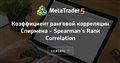Коэффициент ранговой корреляции Спирмена - Spearman's Rank Correlation
• www.mql5.com
Коэффициент ранговой корреляции Спирмена - это непараметрический метод, который используется с целью статистического изучения связи между явлениями. В этом случае определяется фактическая степень параллелизма между двумя количественными рядами чисел. Практический расчет коэффициента ранговой корреляции Спирмена включает следующие этапы: При...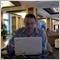Moderator
117467

This indicator for MT4?
Spearman's Rank Correlation - indicator for MetaTrader 4Moderator
117467

After that - I compiled this indicator in MetEditor -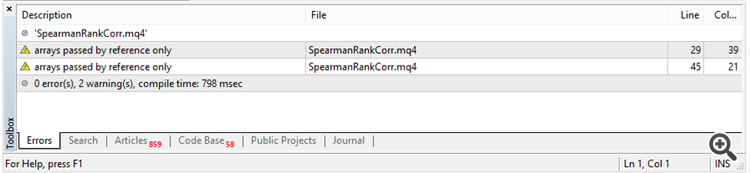After that - I refreshed indicator's folder in Navigator -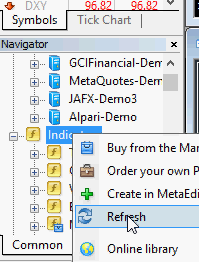and I attached this indicator to the chart - it works: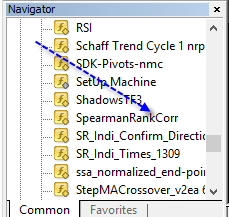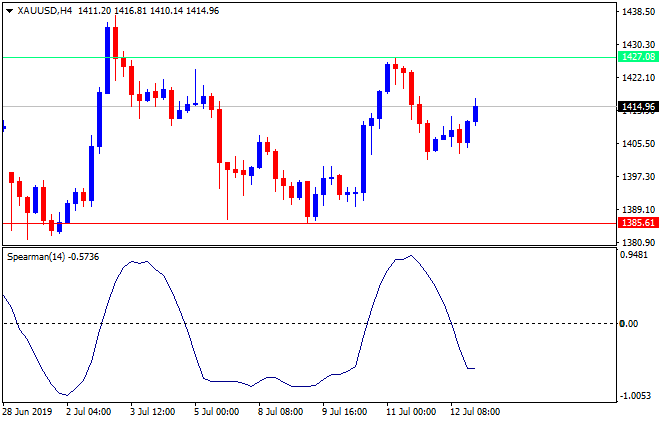122

Sergey Golubev:

After that - I compiled this indicator in MetEditor -

After that - I refreshed indicator's folder in Navigator -

and I attached this indicator to the chart - it works:

no.

i just noticed,the link for the indicator in code is different from the indicator i am talking about

can you please compile the code i have given above?

Here is the indicator , if you want to have a lookModerator
117467

sanders:

no.

i just noticed,the link for the indicator in code is different from the indicator i am talking about

can you please compile the code i have given above?

Here is the indicator , if you want to have a look

I compiled your indicator (indicator from the first post).
Indicator attached.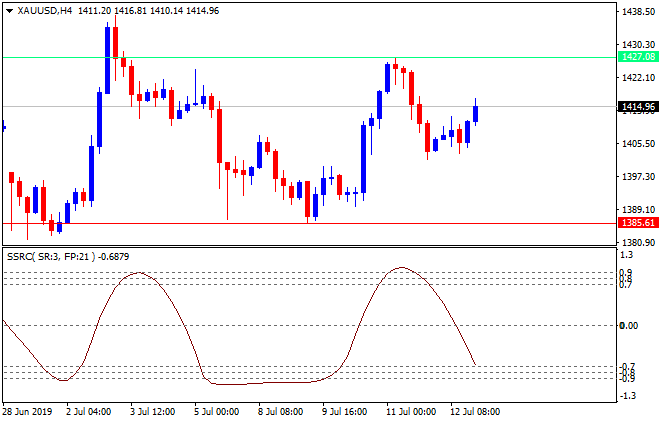Not a problem at all (good indicator).

Files:
SSRC.ex4 21 kb
SSRC.mq4 15 kb122

Sergey Golubev:

I compiled your indicator (indicator from the first post).
Indicator attached.

Not a problem at all (good indicator).

can you please have a look at the video :

https://youtu.be/Cz4ewFFD5i4Moderator
117467

sanders:

can you please have a look at the video :

https://youtu.be/Cz4ewFFD5i4

If you compile the indicator in MetaEditor so the MetaEditor is creating ex4 from mql4 source (compiling = creating ex4/ex5 files).
Because metatrader 4 is using ex4 files only (Metatrader is not using source codes).

So, after compiling indicator in MetaEditor (after MetaEditor creates new ex4 file) so you can attach this new ex4 file to the chart.
And old ex4 file may be disappeared.

It is how I understood your video.

--------------

Means: you should compile and after that - attach to the chart.
because if you attach to the chart and after that - compile so it will not work.